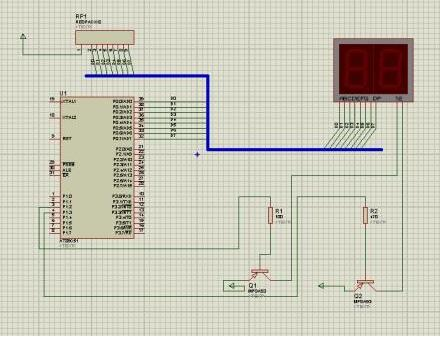51单片机与PC机的串口通讯设计

#include

#defineucharunsignedchar

#defineSLAVE0xF1// 单片机 地址

#defineBN10//传送一次的字节数

ucharidatardata［10］;

ucharidatatdata［10］={“abcdefghij”};

charsend（）;

voidmain（void）{

uchari;

for（i=0;i《10;i++）

TMOD=0x20;//定时器T1定义为模式2

TL1=0xE6;TH1=0xE6;//置初值

PCON=0x00;

TR1=1;

SCON=0xE0;//设置串行口控制字，方式3，允许接收

send（）;//发送数据函数

}

voiderror（void）

{

SBUF=0xFF;//发给PC机数据出错标志

while（TI！=1）;//等待发送完

TI=0;

}

{uchari，p;

while（1）

{

while（RI！=1）;//等待PC机的联络信号

RI=0;

error（）;//若地址出错，发出错标志

else

{

TB8=0;//清地址标志位，准备接收数据

SBUF=0x00;//发约定代码00H，说明地址正确

while（TI！=1）;TI=0;

while（RI！=1）;RI=0;

{

while（1）

{p=0;

for（i=0;i{

while（RI！=1）;RI=0;

rdata［i］=SBUF;// 单片机 接收数据

p=p+rdata［i］;

}

while（RI==0）;RI=0;

if（SBUF==p）

{SBUF=0x00;//校验和相同，发0x00给PC机

while（TI！=1）;TI=0;

}

else

{SBUF=0xFF;//校验和不同，发0xFF给PC机

while（TI！=1）;TI=0;

}

}

TB8=1;//重置地址标志位

return（0）;

}

}

}

}

charsend（）// 单片机 发送数据函数

{

uchari，p;

do{

p=0;//清校验和

for（i=0;i{SBUF=tdata［i］;//发送数据

p=p+tdata;//计算校验和

while（TI！=1）;TI=0;

}

SBUF=p;//发送校验和给PC机

while（TI！=1）;TI=0;

while（RI！=1）;RI=0;

}while（SBUF！=0）;//PC机接收不正确， 单片机 重新发送

TB8=1;//重置地址标志位

return（0）;

}

• 没有资料